# A giraffe

A giraffe can run up to 46.93 feet per second. How far could a giraffe run in 1.8 seconds?

s =  84.474 ft

### Step-by-step explanation: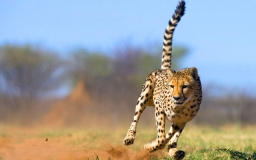Did you find an error or inaccuracy? Feel free to write us. Thank you!Tips to related online calculators
Do you want to convert length units?
Do you want to convert velocity (speed) units?
Do you want to convert time units like minutes to seconds?

## Related math problems and questions:

• Thunderstorm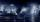The sound travels 1 km in about 3 seconds. How far is the storm if there is a time interval of 8 seconds between lightning and thunder?
• Walking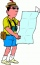Lucie can walk about 3 4/5 miles each hour. How far can she walk in 2 hours 45 minutes?
• Cyclist 9A cyclist travels at a speed of 4.25 km per hour. At that rate, how far can he travel in 3.75 hours?
• RunningJoanne ran 8 1/2 Kyleigh 1 2/3 and John 6 3/4 miles in one day. How many miles did the girls run in 3 days?
• Thunder and lightning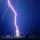There was a glimpse of the sky, and we hear thunder in 4.5 seconds. The light spreads at 300,000 kilometers per second so that we can assume the flash instantly without delay. However, the sound's speed is much lower in the air, one-third of a kilometer p
• The caravans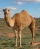At 6:00 in the morning, a caravan set out of the oasis at a speed of 1.5 miles per hour. Two hours later, a second caravan followed her at 2.5 miles per hour. When did the faster caravan run slower? How far from the oasis?
• Cindy runSuppose it takes 1/3 of an hour for Cindy to run 3/5 of a mile. How many miles does she run in 1 hour?
• Aircraft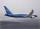If an airplane flies 846 km/h for 8 h, how far does it travel?
• Earth and Sun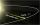Earth is 150 million km away from the Sun. 300,000 km of sunlight travels in 1 second. How long will the light come from the Sun to the Earth?
• Car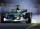Car is traveling 98 km per hour. How long-distance it travel in 1 minute?
• A raindrop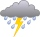A raindrop falls at a rate of 9.3 meters per second (m/s). Use the following facts to convert this speed to kilometers per hour (km/h). 1 km = 1000 m 1 min = 60 sec 1 hour = 60 minThe railroad runs parallel to the railway. The train travels at 36 km/h on track. In the opposite direction, the motorcycle rides at 90 km/h. The train passes in 3 seconds. How long is a train?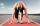Marian runs 12 meters in 8 seconds. How far would Marian run for 70 seconds if he still runs at the same pace?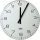The Nürburgring in Germany is a racetrack that hosts racing events all year. Two of the fastest laps ever driven on the track are 6 minutes 47.30 seconds and 6 minutes 52.01 seconds. How much faster is the 6 minutes 47.30 seconds time than the 6 minutes 5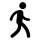Reeta walks 5/7 km in 1 hour. How far does she go in 3 1/2 hours?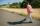Dana started at 10:00 from point A to point B. These points are distant 12 km. Determine how fast Daniel went when place B arrived at 11:54. Speed express in km/h.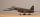The average speed of sound is 330 meters per second. Estimate how long it will take to hear the church bell 1 km away. Calculate the distance from what would hear sound after 10 seconds.